U.S. Dept. of Commerce / NOAA / OAR / PMEL / Publications

## Variability of the sea surface temperature in the eastern equatorial Pacific during 1986-88

### (Mixed Layer Temperature Variability, continued)

#### Vertical Diffusive Heat Flux

Recent observational studies of near equatorial turbulence at 140░W [Gregg et al. 1985; Peters et al., 1987; Moum et al., 1989] indicate that the turbulent heat flux at the base of the mixed layer is similar in magnitude to the atmospheric heat flux at the ocean surface. Turbulent processes are not directly measured by the equatorial moorings; hence the turbulent heat flux can be only estimated using a parameterization of the mixing processes. We assume that the turbulent heat flux is down the temperature gradient and represented by an eddy coefficient, K:(10)

Relating the eddy coefficient to a single parameter of the mean field provides the simplest parameterization of turbulent mixing. Following Pacanowski and Philander , we assume that Kis a function of the Richardson number Ri and write:(11)

with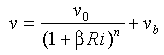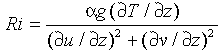where n,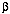,, K, andare empirical constants, g = 9.8 m s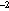is the gravitational acceleration, and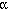= 8.75 ū 10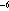(T+9) (░C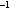) is the thermal expansion coefficient. The temperature T is in degrees Celsius.

The parameterization in (11) is the form of vertical mixing used in several recent numerical general circulation model simulations of the tropical oceans [Philander, 1990]. Peters et al.  have shown on the basis of turbulence measurements at 140░W that this functional form for K(Ri) yields reasonable agreement with equatorial measurements for Ri > 0.5; however, the empirical constants in (11) derived from the data differ in magnitude from those used in the numerical models. These constants are likely to vary with location and time as well as with the vertical resolution over which the gradients are calculated. Hence for our study we assumed the constants given by Pacanowski and Philander : n = 2,= 5,= 5 ū 10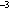ms,= 1 ū 10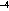ms, and K= 1 ū 10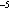ms.

The vertical diffusive heat flux was computed from the mooring data in Figure 5 using (10) and (11). Vertical gradients of temperature and velocity were estimated by centered differences on the 5-m gridded data. Figure 7 shows the mixed layer depth and the Richardson number below the mixed layer. The seasonal variations of the Richardson number was similar in 1986 and 1987; Ri was relatively high in August-September and low in May-June. The small Ri in May-June is clearly associated with the occurrence of strong shear below the mixed layer as the Equatorial Undercurrent (EUC) intensifies. The high Ri in August-September corresponds to the deepening of the EUC and the reduction in near surface shear. When the mixed layer is deep the Richardson number tends to be large. However, in March-April 1988 the largest Richardson numbers occurred at a time when the mixed layer was very shallow. At that time the near-surface shear was small and the vertical temperature gradient was large as cool surface water appeared in the eastern equatorial Pacific.Fig. 7. Low-pass-filtered (91-day Hanning filter) time series of the mixed layer depth h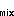and Richardson number Ri at the base of the mixed layer at 0░, 110░W, estimated as described in the text.

The turbulent diffusion of heat out of the mixed layer is shown in Figure 6d. As can be expected, the variations in Ri have a strong influence on the estimated heat flux. High Ri corresponds to small vertical heat flux, and vice versa. Seasonal changes in mixed layer temperature are well correlated with the vertical heat flux except in spring 1988. In fall 1986 and spring 1987, the large mixed layer heating corresponded to decreased turbulent flux out of the mixed layer. Similarly, the decreased heating in November-December 1986 and May 1987 corresponded to increased downward turbulent flux. Spring 1988 remains anomalous, since the high Ri at that time suppresses the turbulent flux; vertical heat flux out of the mixed layer decreased at the same time that the layer was cooling.

On average, the vertical turbulent diffusion exported about 130 W mto the deep ocean with rms fluctuations of 40 W m. This flux nearly balanced the net surface flux (115 W m) into the mixed layer (Figure 4), suggesting that vertical diffusion is probably the dominant internal oceanic process controlling the annual average mixed layer temperature in the eastern Pacific.

Go back to previous section or forward to next section

PMEL Outstanding Papers

PMEL Publications Search

PMEL Homepage